# Volumetric analysis

Basically, there are two types of chemical analysis:

• Qualitative analysis
• Quantitative analysis.

Qualitative analysis tells ‘what’ is in a sample, while quantitative analysis is used to tell ‘how much of a given component is in a sample.

## Volumetric analysis

Volumetric analysis is one of the quantitative methods of analysis which basically involves the determination of the quantity of a substance present in a given solution by reacting a known volume of it with a solution of another substance of known concentration.

The process by which this analysis is carried out is called ‘titration’.

### Equivalent weight

The equivalent weight of an element is defined as the number of parts by weight of that element which either combines or displaces 1.008 parts by weight of hydrogen or 8 parts by weight of oxygen or 35.5 parts by weight of chlorine.

Mathematically :

1. Equivalent weight of an element :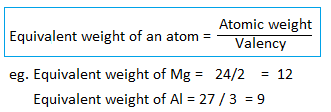2. Equivalent weight of an acid :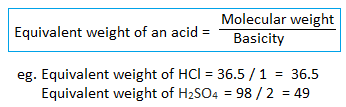3. Equivalent weight of a base :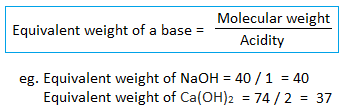Note :

• Basicity – Number of replaceable hydrogen ions present in acid.
• Acidity – Number of replaceable hydroxyl ions present in base.
• The basicity of CH3COOH is 1 (not 4) i.e. no. of replaceable hydrogen only (not all hydrogen).

4. Equivalent weight of salt :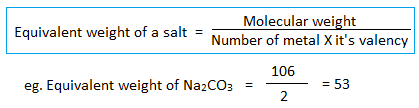5. Equivalent weight of oxidizing and reducing agent :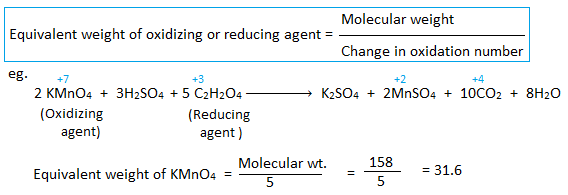### Gram equivalent weight

Note: Equivalent weight of any substance is simply a number. It does not have any units.

The equivalent weight expressed in gram is called gram equivalent weight.

Eg. one gram equivalent weight of NaOH is 40 grams of NaOH. Similarly, the two-gram equivalent weight of NaOH is 80 grams.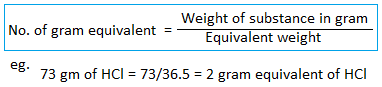### Different ways of expressing the concentration (strength) of solution

The amount of a solute present in the definite quantity of a solution is called the concentration of a solution. The concentration of a solution may be expressed in any of the following ways :

1. Gram per literThe amount of solute in gram present in one liter of solution is known as gram per liter of solution.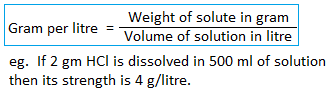2. NormalityThe number of gram equivalent of solute present in a liter of solution is known as normality of solution.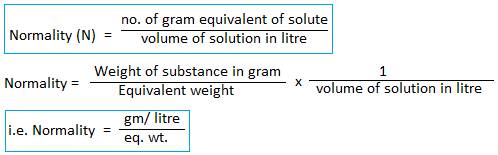Normal solution(N)A solution containing one gram equivalent of solute in one liter of solution is known as a normal solution. For example, if 53gm of Na2CO3 is present in one liter of the solution then its strength is 1N and is said to be a normal solution.

Semi-normal solution(N/2)A solution containing half of the gram equivalent of solute in one liter of solution is known as a semi-normal solution. For example, if 26.5gm of Na2CO3 is present in one liter of the solution then its strength is N/2 and is said to be a semi-normal solution.

Decinormal solution(N/10)A solution containing 1/10th of gram equivalent of solute in one liter of solution is known as a decinormal solution. For example, if 5.3gm of Na2CO3 is present in one liter of the solution then its strength is N/10 and is said to be the decinormal solution.

Centinormal solution(N/100)A solution containing 1/100th of gram equivalent of solute in one liter of solution is known as a centinormal solution. For example, if 0.53gm of Na2CO3 is present in one liter of the solution then its strength is N/100 and is said to be centinormal solution.

3. MolarityThe number of moles of solute present in a liter of solution is known as the molarity of a solution.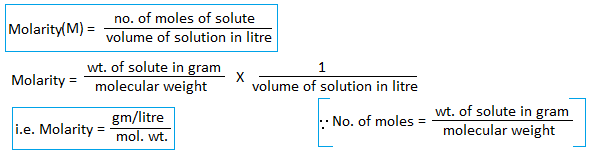Molar solution(1M): A solution containing one mole of solute present in one liter of solution is known as a molar solution. For example, if 106g of Na2CO3 is present in one liter of the solution then its strength is 1M and is said to be a molar solution.

### Relation between normality and molarity

4. Molality : The number of moles of solute present in one kilogram of solvent is known as molality of solution.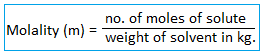Molal solution(1m) : A solution containing one mole of solute present in one kilogram of solvent is known as molal solution. Example, if 106g of Na2CO3 is present in one kilogram of solvent then its strength is 1m and is said to be molal solution.

5. Percentage (%) : Percentage strength means number of parts of solute in 100 parts of solvent. It is expressed in three different ways: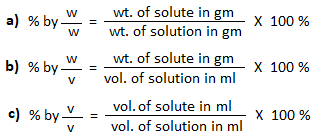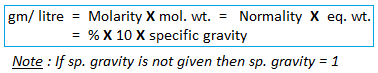### Normality equation (N1V1 = N2V2)

Q) How much NaOH is required to prepare 250 ml of N/10 NaOH solution?

Amount of NaOH required (w) = NEV/100

= 0.1 X 40 X 250 / 1000 = 1 gm.

Hence, 1gm NaOH is dissolved in 250ml water to prepare N/10 solution.

Normality factor (f) : Normality factor is defined as the ratio of actual weight of substance taken to the theoretical weight of substance to be taken.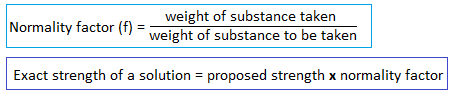### Titration

The process of determining the strength of unknown solution with the help of standard solution (i.e. the solution whose strength is known) is called titration.

Typically, the titrant (the solution of known strength) is added from a burette to a known quantity of the titrand (the unknown solution) until the reaction is completed.

Titration is also called standardization.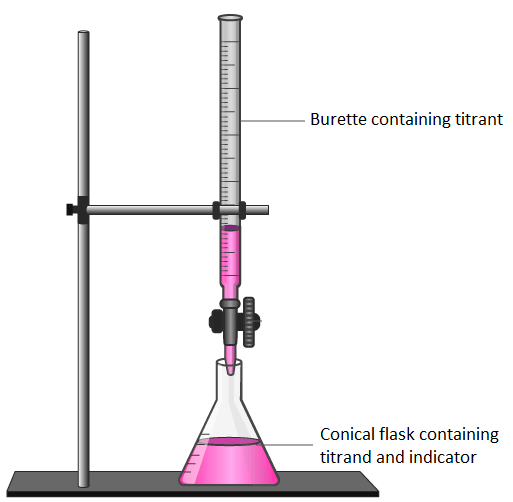Titration can be classified into following types:

• Acid-base or neutralization titration
• Oxidation-reduction or redox titration
• Precipitation titration
• Complexometric titration

Acid-base or neutralization titration :

The titration which involves the acid-base reaction( i.e. neutralization reaction) is called acid-base titration.

Acidimetry: The process of determining the strength of unknown acid by titrating it with the standard solution of alkali in presence of indicator is called acidimetry.

Alkalimetry: The process of determining the strength of unknown base by titrating it with the standard solution of acid in presence of indicator is called alkalimetry.

Oxidation-Reduction or Redox titration:

The process of determining the strength of an oxidizing agent by titrating it with the standard solution of reducing agent or vice-versa is called redox titration. For example:

Titration of potassium permanganate (KMnO4) (i.e. oxidizing agent) with standard oxalic acid (C2H2O4) (i.e. reducing agent) in acidic medium is a redox titration.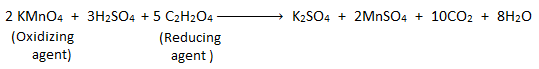Precipitation titration:

The titration in which a precipitate is formed as a result of reaction between the reacting solutions is called precipitation titration. For example: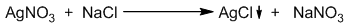### Some terms and terminology related to volumetric analysis

Titrant (Standard solution) : The solution whose strength is known during the titration is known as titrant. The titrant is taken in a burette during titration.

Titrand : The solution whose strength is not known i.e. the solution whose strength is to be determined during titration is known as trand. The titrand is pipette out by the pipette and taken in the flask during titration.

IndicatortsIndicators are those chemical substances which indicate the completion of reaction (i.e. end point) during titration by changing their own colour. Indicators are of two types:

1. Internal indicators
2. External indicators

Internal indicatorsThe indicator which is added to the flask is known as internal indicator. Eg. acid-base indicators, self indicators, precipitation indicators, etc.

Acid-base indicators: Indicators used in acid-base titration are called acid-base or pH indicators. Eg. methyl orange, phenolphthalein, litmus paper, methyl red, etc.

Self indicators: A self indicator is a rectant which itself indicates the end point of reaction by changing the color of reaction mixture. Eg. KMnO4 acts as an self indicator during titration between KMnO4 and oxalic acid because it gives pink colour in basic medium and colourless in acidic medium.

External indicators: External indicator is added in between the titration process by taking a drop of titrated mixture.

Example: potassium ferricyanide is used in titration of ferrous ammonium sulphate and K2Cr2O7.

End point : The stage(point) during the titration at which indicators indicates the completion of reaction by changing its own colour is called end point.

If the titration is carried out in between acid and base then the end point is called neutral point.

Equivalence point (Theoretical end point)The stage (point) during the titration at which number of gram equivalent of titrant is equal to the number of gram equivalent of titrand is called equivalence point.

Indicator changes its colour after the equivalence point, hence equivalence point is also called theoretical end point.

Titration error In theoretical concept, when equivalent amount of titrant is added ti the titrand the indicator should change the colour and indicate the end point. But in actual practice, the indicator changes its colour either in acidic or alkaline medium. Therefore in practice a small difference occurs between equivalence point and end point. This difference between end point and equivalence point is called titration error.

### Primary and secondary standard solution/substance

Primary standard solution/substance:

The substance whose standard solution can be prepared directly by dissolving the known weight of substance in fixed volume of solution is known as primary standard substance. Examples: anhydrous sodium carbonate (Na2CO3), oxalic acid (C2O4H2), silver nitrate (AgNO3), etc.

The solution of primary standard substance is known as primary standard solution. Concentration of primary standard solution remains constant for certain interval.

Criteria for a substance to be primary standard:

For a substance to be a primary standard it must fulfill the following requirements:

• It must be available in pure form and should be non-toxic.
• It should be stable (i.e. composition should not be vary for sufficiently long time).
• It should not be hygroscopic or deliquescent.
• It should have high molecular or equivalent weight.
• It should be easily soluble in water and not decomposed by water.

Secondary standard solution/substance:

The substance whose solution can be standardize or strength can be determined by the help of primary standard solution is known as secondary standard substance. Examples: HCl, NaOH, FeSO4, KMnO4, etc.

The solution of secondary standard substance is known as secondary standard solution. Concentration of secondary standard solution changes after certain interval.

### Selection of indicators during acid-base titration

Indicators are the chemical species that indicates the end point by changing its own colour. Each pH indicator has its own pH range for its colour change. In order to determine the accurate end point of acid-base titration, the indicator should be selected in such a way that the pH range for the colour change of the indicator must coincide with the pH change (jump) at the end point of reaction.

Mainly two types of indicators i.e. methyl orange and phenolphthalein are used during acid-base titration. Methyl orange has pH range 3.1-4.4 and phenolphthalein has 8.2-10.

There are four types of acid base titrations.

1.Strong acid vs strong base titration:

The indicators pH range of both methyl orange (i.e. 3.1-4.4) and phenolphthalein (i.e. 8.2-10) coincide with the pH jump (i.e. 3-11) at end point. Hence, either methyl orange or phenolphthalein can be used as indicator during strong acid vs strong base titration.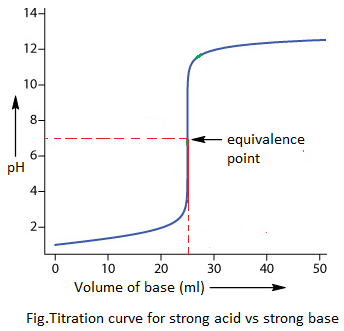2. Strong acid vs weak base titration:

The indicators pH range of only methyl orange (i.e. 3.1-4.4) coincides with the pH jump (i.e. 3-8). Hence, only methyl orange can be used as indicator during strong acid vs weak base titration.3. Weak acid vs strong base titration:

The indicators pH range of phenolphthalein (i.e. 8.2-10) only coincides with the pH jump (i.e. 6-11). Hence, only phenolphthalein can be used as indicator during weak acid vs strong base titration.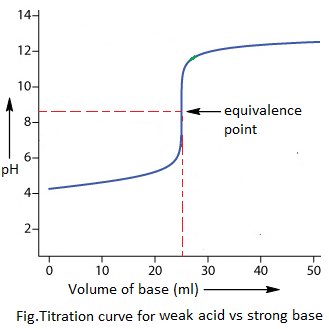4.Weak acid vs weak base titration:

The indicators pH range of neither methyl orange (3.1-4.4) nor phenolphthalein (i.e. 8.2-10) coincides with the pH jump ( 6-8). Hence, weak acid vs weak base cannot be usually titrated due to lack of suitable indicators.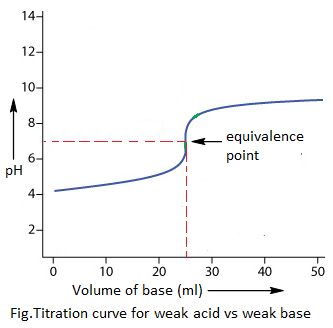Note :

Strong acid – HCl, HNO3, H2SO4, etc.

Strong base – NaOH, KOH, Ca(OH)2, etc.

Weak acid – formic acid, acetic acid, oxalic acid, etc.

Weak base – NH4OH, Cu(OH)2, Fe(OH)3, etc.

### Numerical problems

1) Find the normality of 0.53 gm/litre Na2CO3 solution.

2) Calculate the normality and molarity of 5% of NaOH solution.

3) What is the normality of 20cc of 2M phosphoric acid (H3PO4) ?

4) Which one has higher concentration and why?

i. 80 g/lit NaOH solution and 3M NaOH solution.

ii. 5.3 g/lit Na2CO3 and N/10 Na2CO3 solution.

5) 20 cc of 2N HCl is completely neutralized by 1.5N NaOH. Calculate the volume of base.

6) X cc of 5N HCl was diluted to one litre of normal solution. Calculate the value of X.

7) What volume of water must be added to 40 ml of 0.25N acid solution in order to make it exactly decinormal solution?

8) 4 gm H2SO4 is mixed with 50 ml 2N NaOH solution. Predict the solution is either acidic or basic or neutral.

9) 40 ml 2N H2SO4 is mixed with 20 ml 2N NaOH and 20 ml 1.5N Na2CO3. Predict the solution is either acidic or basic or neutral.

10) 50 ml 2N H2SO4 is mixed with 30 ml 3N NaOH. Predict the solution is either acidic or basic or neutral. Also calculate the resulting strength of solution.

11) 25 cc of a solution of HCl reacts completely with 10 gm of CaCO3. What is the strength of HCl in normality?

12) 100 cc of 0.1 N HCl is mixed with 50 cc 0.1 N NaOH. Calculate the concentration of resulting solution in normality, molarity and gram per litre.

13) 20 ml 2N HCl is mixed with 35ml 1N NaOH. The mixture is then diluted to 1 litre. Calculate the resulting normality of diluted solution.

14) 25 cc of an alkali solution is mixed with 8 cc of 0.75N acid solution and for complete neutralization it further requires 15 cc of 0.8N acid solution. Find the strength of the given alkali solution.

15) 20 ml of a decinormal solution of NaOH neutralizes 25 ml of solution of dibasic acid containing 6gm of acid per litre. Find the equivalent weight and molecular weight of acid.

16) 0.012 gm of a divalent metal is completely dissolved in 40 cc of N/10 NaOH for complete neutralization. Find the equivalent weight and atomic weight of metal.

17) 3 gm trivalent metal was completely dissolved with 750 ml of 1N HCl. The residual solution further required 1000 ml of N/2 NaOH solution for complete neutralization. Find the atomic mass of the metal.

18) How many moles of H2SO4 are required to neutralize 4 litre of 2N NaOH solution?

19) 0.715 gm of Na2CO3.xH2O required 20 ml of seminormal HCl solution for complete neutralization. Find the value of x.

20) 1 gm of NaOH is added to 2 litre of H2SO4 solution so that the pH of resulting solution is 7. Find the molarity of acid solution.

21) 0.8 gm of a divalent metal was dissolved in 100 cc of 1.28 normal HCl and the solution was diluted to 200 cc. 50 cc of this diluted solution required 54.6 cc of 0.22 N NaOH for neutralization. Calcute the atomic mass of the metal.

22) x gm of metal having equivalent weight 12 was completely disssolved in 100 cc of seminormal HCl the volume was then made upto 500 cc. 25cc of this acid required 17.5 cc of N/10 NaOH for complete neutralization. Find the value of x.

23) What volume of 12N HCl and 3N HCl must be mixed to form one litre 6N HCl?

24) What volume of 12M NaOH and 2M NaOH should be mixed to get 2 litre of 9M NaOH solution.

25) 1.42gm of a mixture of calcium and magnesium carbonates were completely dissolved in 200 cc of N/5 HCl. The solution was made upto 250cc and 10cc of this solution required 12cc of N/30 Na2CO3 solution for neutralization. Calculate the percentage composition of the mixture.

26) 1 gm of an ordinary sample of limestone dissolved in 16.6cc of 0.92N HCl leaving some sandy residue. calculate the percentage of pure CaCO3 in the sample.

Reference: https://chemicalnote.com/

Reactions